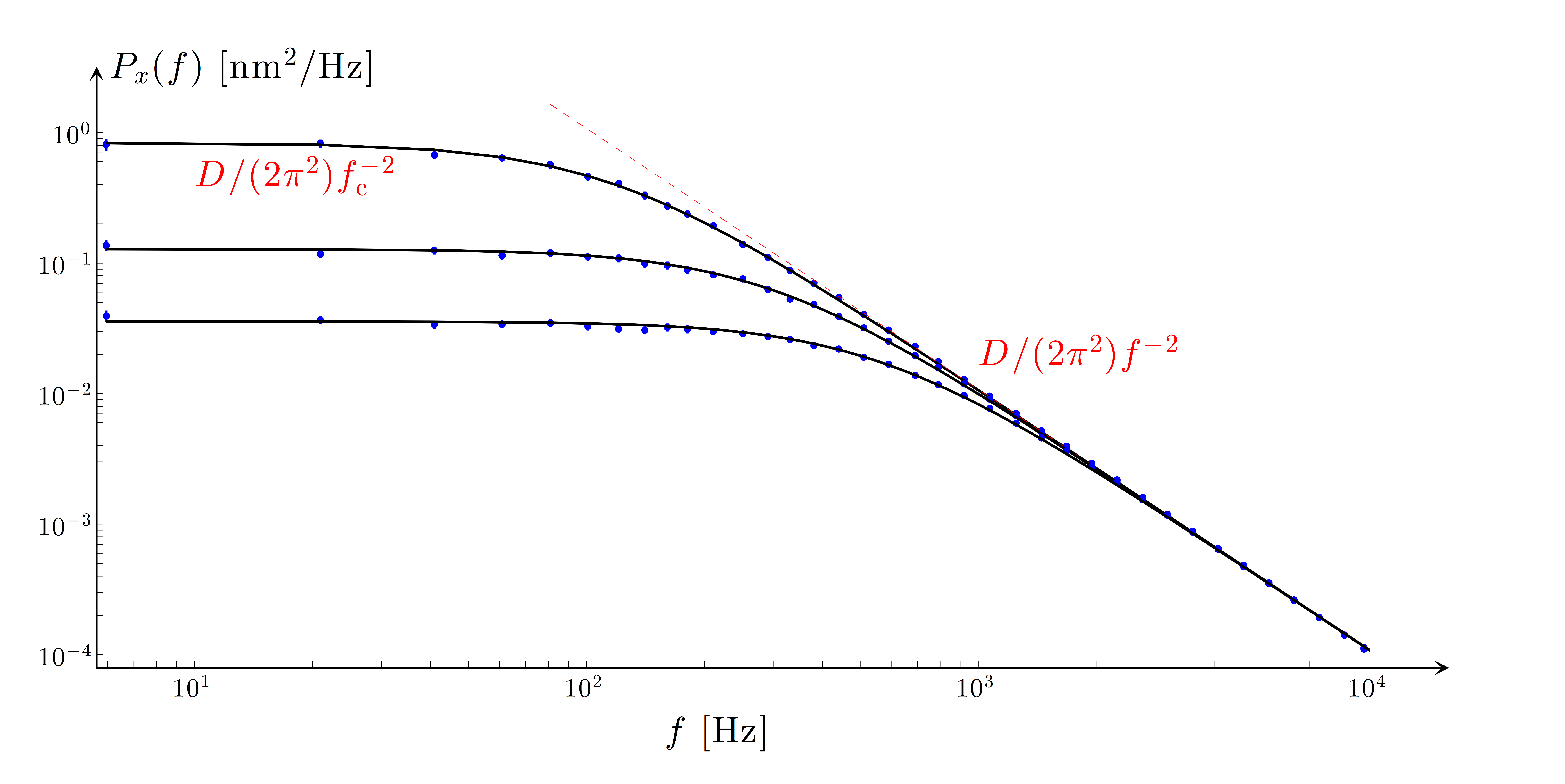# Figure 9.14 — Power spectrum analysisFigure 9.14 — Power spectrum analysis. Power spectral densities (PSDs) corresponding to a polystyrene sphere (a = 1.03 μm) optically trapped with 2.3, 6.0 and 10.8 mW laser power (λ0 = 1064 nm) at the sample. The symbols and error bars are the experimental PSDs [Eqs. (9.43)] and the solid lines are the theoretical PSDs [Eq. (9.39)] corresponding to the fitted parameters. The fitted values of the corner frequency are fc,x = 114 ± 2, 290 ± 3 and 550 ± 5 Hz (corresponding to κx(ex) = 13.9 ± 0.2, 35.4 ± 0.4 and 67.1 ± 0.6 fN/nm) and those of the unit conversion factor [Eq. (9.31)] are Sx(ex) = 483, 192 and 107 nm/V.